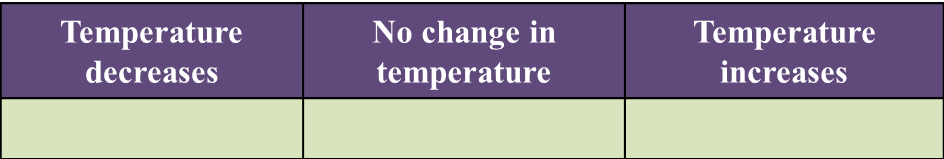Question 1:
Diagram 1.1 and Diagram 1.2 show an experiment to study the heat change in chemical reactions at room temperature.(a) Based on Diagram 1.1, what is your observation about the change in temperature?(b) What is the final reading for the thermometer in Diagram 1.2? [1 mark]

(c) State the variables in this experiment.
(i) Constant variable [1 mark]
(ii) Responding variable [1 mark]

(d) State one hypothesis for this experiment. [1 mark]